# Excels INDIRECT Function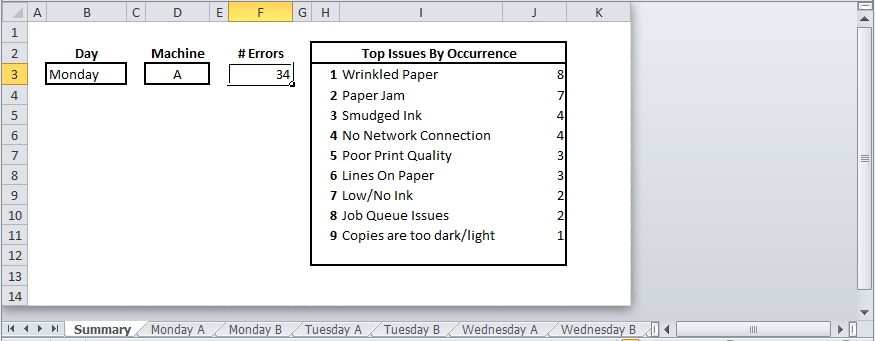The INDIRECT “function returns the reference specified by a text string”.  What does this mean and why would I use it?

I have seen people have problems with amending formula to reference different sheets in particular, most of them just recreate the formula rather than trying to amend it.

One of the ways around this that I’m going to show you now is to use the INDIRECT Function.

The advantage I find with INDIRECT is that once set up it allows you to quickly query different sheets or ranges depending on certain criteria without having to amend your formula.

## INDIRECT Function Example

An example of where this might be used could be in a file such as this:There is a summary sheet with a drop-down list for the day of the week and also a machine name.

Depending on the day and machine name, the formula in the rest of the sheet will query the relevant Day/Machine sheet and return the top issues by frequency.

In the image above, the day is Monday and the machine is A, so, using the INDIRECT function Excel will query the sheet Monday A.

If the day is changed to Wednesday and machine is B, then without having to amend any formula, Excel will query the sheet Wednesday B.

Above is a simplified example of where this might be used just for demonstration.

In reality if you had 2 machines and 5 working days in the week then a Summary sheet with 10 tables wouldn’t take too long, but the cases where i have used this formula and shown it to others there were a lot more combinations of sheets or ranges to be queried and the INDIRECT function was a big time saver going forward.

## INDIRECT Demonstration

### File Set-Up

A demonstration of how the function works might explain it easier.

I have set up a file with 5 sheets as follows:

• “Demo” is where I am going to demonstrate the INDIRECT function
• “Sheet2” is the default name for the second sheet of my file
• “Sheet 3”, note there is a space between the word Sheet and number 3
• “AnotherSheet” is some other non-default sheet name, but with no spaces
• “Another Sheet” is another non-default sheet name, but this does contain spaces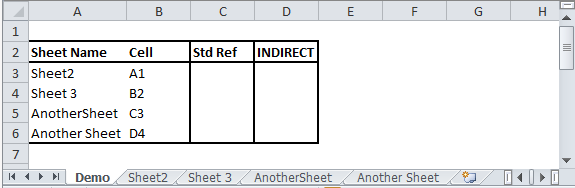Within each sheet, I will enter “Hello ” followed by the cell reference in column B of the table above and i will enter this only in the cell reference for each sheet.

So, Sheet2 will only contain “Hello A1” in cell A1 and Sheet 3 will only contain “Hello B2” in cell B2.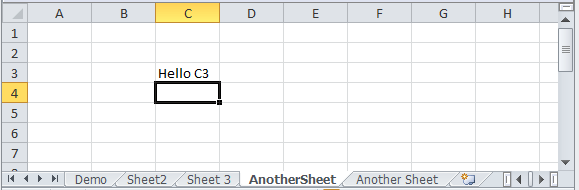### Standard Reference

On the Demo sheet, if we wanted to reference cell A1 of Sheet2, or cell B2 of Sheet 3 we would just type “=” and then click on the relevant sheet/cell.

And that is exactly what we will do for cells C3 to C6 of the Demo sheet.

The formula for C3 should be “=Sheet2!A1” and for C4 it should be “=’Sheet 3′!B2”.

Apart from the obvious sheet name and cell reference being different, did you spot a difference?

Where a sheet name contains a space, the sheet name will be contained between single quotes/apostrophes.

Look at the formula in C5 and C6 which reference AnotherSheet and Another Sheet.

If there is a space you need to include quotes in your reference.

This is something you will need to remember when creating an INDIRECT function that will reference other sheets.

### INDIRECT Function

Now that we have our standard reference out of the way, lets create some INDIRECT functions that will be a bit more flexible.

Let’s start in cell D3.

• =INDIRECT( we start our INDIRECT function and the sheet/cell reference we are going to create will be contained within brackets
• A3&”!”& the first part of our string is the sheet name which is in cell A3.  The sheet name reference will need to include an exclamation mark at the end before going to the cell reference.  So here we are saying Sheet2(A3) and then and exclamation mark (&”!”) and then we move to the cell reference (&)
• B3) is the cell reference we are querying and then we close the INDIRECT function with a right bracket.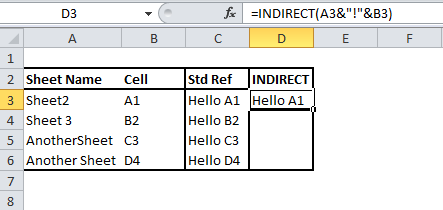This formula gives us the same result as C3.

If you tried copying that formula to D4 however, it will not work and that is because our sheet name in A4 contains a space, so our INDIRECT function will have to allow for that.

In D4, enter a similar function, but this time with a single quote/apostrophe before and after the sheet reference.

• =INDIRECT( again we open our INDIRECT function
• “‘” our first single quote/apostrophe is contained between two double quotes (with spaces, this is ” ‘ “)
• &A4&”‘!” after the first quote we then add the sheet name from A4 (&A4) and we end the sheet name with the second single quote and exclamation mark (&”‘!” – with spaces that is ” ‘ ! “)
• &B4) and then we add our cell reference and close the function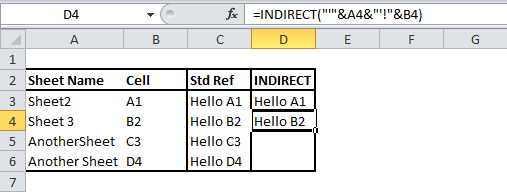If you copy the formula in D4 to cells D3 to D6, you will see it works – even though not all sheet names in column A contain spaces.

### INDIRECT Next Example

Let’s take our INDIRECT example a step further and make it a bit more useful than it has been so far in our demonstrations.

On the Demo sheet, I will change A8 to B9 as follows

• A8 – “Pick A Sheet:” header
• A9 – This is where we will be entering a sheet name
• B9 – This is where we are about to create our INDIRECT function

Let’s break up or INDIRECT funtion in cell B9 into two parts:

1. =INDIRECT(“‘”&A9&”‘!”& we start the INDIRECT function.  Our sheet name which will be entered in A9 is preceeded by a single quote and succeeded with a single quote and exclamation mark.  We then get ready to move onto the cell reference.
2. VLOOKUP(A9,A3:B6,2,0)).  Our cell reference is going to be the result of a formula in this case.  We are going to look up the sheet name in cell A9 and retrieve the relevant cell reference from the A3 to B6 range.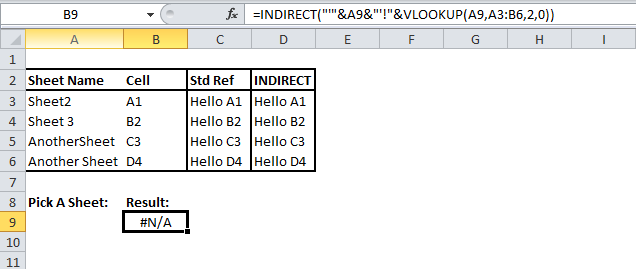Initially we will get a #N/A error message, but this will change when you enter a sheet name (I suggest copying a sheet name from A3 to A6).Whenever you enter a sheet name in A9, the function in B9 will put together the relevant sheet/cell reference text and make it a usable reference that Excel understands.

In a future post I will use an example of a large file to demonstrate how several different functions, including INDIRECT can come together to be extremely useful, before then, there are a few more topics that I would like to cover.

In the meantime, I hope you find this post informative and the function useful.

Follow